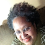## Wednesday, 7 October 2020

### Science Questions Series || Class 7 || Chapter - Light

This worksheet is from chapter Light of class 7. This worksheet will help the student in checking their knowledge of this chapter.

1. A ________ changes the direction of the light that falls on it.
2. The change in direction of light by a mirror is called ________ of light.
3. The objects having polished, shining surfaces reflect more ________than objects having unpolished dull surfaces.
4. The ray of light which falls on an object is called ________.
5. The smooth surface of still water can also act as a ________
6. The ray of light which is sent back by an object is called ________.
7. The image which can be obtained on a screen is called a ________ image.
8. We can see the objects around us because the reflection of  light received from a ________object into our eyes.
9. The ________ image is just an illusion.
10. The image which cannot be obtained on a screen is called________ image.
11.  It is not possible to obtain a virtual image on the ________.
12. The image in a ________ mirror is of the same size as the object.
13. The distance of image from the mirror is ________ to the distance of object from the mirror.
14. The image formed in a plane mirror is ________ and ________.
15. Any polished and shining ________ can act as a mirror.
16. A ________ mirror is that mirror whose reflecting surface is the part of a hollow sphere of glass.
17. A spherical mirror are of two type ________ and ________ mirror.
18. When the parallel ray of light spread out, we say that the rays of light are ________.
19. Depending on the distance of the object, concave mirror forms mainly two kinds of images: ________ and ________.
20. A ________ mirror is that spherical mirror in which the reflection of light takes place at the concave surface.
21. A ________ mirror is that spherical mirror in which the reflection of light takes place at the convex surface.
22. The distance from the focus up to the concave mirror is called the ________ of concave mirror.
23. Concave mirror is also known as a ________ mirror.
24. When an object is placed close to a concave mirror is ________, ________, and ________ then the object.
25. A ________ is a piece of transparent glass bound by two spherical surface.
26. The lens which is thicker in the middle than at the edges is called a ________ lens.
27. The lens which is thinner in the middle than at the edges is called a ________ lens.
28. The focus of a convex lens is a point where  ________ rays of light coverage after passing through the convex lens.
29. In the year 1665, ________ discovered by his experiments with glass prisms that white light consist of a mixture of lights of seven colours.
30. The ________ consists of seven colours.

Other Science worksheets

1.2.